# Shapes and Angles

## Objective

Measure angles that are less than 180°. Distinguish between angle measure and length measurement.

## Common Core Standards

### Core Standards

?

• 4.MD.C.5 — Recognize angles as geometric shapes that are formed wherever two rays share a common endpoint, and understand concepts of angle measurement:

• 4.MD.C.6 — Measure angles in whole-number degrees using a protractor. Sketch angles of specified measure.

## Criteria for Success

?

1. Understand an arc to be a part of the perimeter of a circle.
2. Distinguish angle measure from length measure—i.e., understand that an angle’s measure is independent of the length of its arc or its rays/arms/sides.
3. Estimate the measurement of angles based on their proximity to various benchmark angles (45°, 60°, 90°, etc.).
4. Measure angles less than 180° with protractors, placing the middle notch on the vertex of the angle, aligning a side with the zero or base line on the protractor, and reading the number the second side length touches (MP.5, MP.6).
5. Measure angles with short rays by extending the rays (which can be done since the length of the rays does not determine the angle measure).
6. Construct viable arguments and critique the reasoning of others regarding the reasonableness of an angle measurement by comparing the estimated measurement based on benchmark angles to the actual measurement (MP.3).
7. See that angle measure is the same regardless of what protractor is being used to measure.

## Tips for Teachers

?

• Students will need two paper circles of different sizes in this lesson. One can be another copy of the paper circles used in previous lessons, e.g., parchment paper baking circles. The second paper circle can be parchment baking sheets of a different size or can be made from tracing a circular object like a cup on any piece of paper and cutting along the tracing.
• Students will need a protractor for the Problem Set. It is recommended that you have students estimate all of the angle measures first, and then hand them a protractor when they are done. This will help students build visual models of benchmark fractions.
• Students will need a protractor and the differently sized circles from Anchor Task #1 for tonight’s homework. If you do not want students to bring them home, the Homework can be cut.

### Lesson Materials

• Paper circle (2 per student) — Each student's circles need to be different sizes
• Markers or crayons (1 per student)
• Circular protractor (1 per student)

#### Fishtank Plus

• Problem Set
• Student Handout Editor
• Vocabulary Package

?

### Problem 1

Fold Circle A and Circle B as you would to make a right-angle template. Trace the folded perpendicular lines. How many right angles do you see at the center of each circle?  Did the size of the circle matter?

#### References

EngageNY Mathematics Grade 4 Mathematics > Module 4 > Topic B > Lesson 6Application Problem and Concept Development

Grade 4 Mathematics > Module 4 > Topic B > Lesson 6 of the New York State Common Core Mathematics Curriculum from EngageNY and Great Minds. © 2015 Great Minds. Licensed by EngageNY of the New York State Education Department under the CC BY-NC-SA 3.0 US license. Accessed Dec. 2, 2016, 5:15 p.m..

Modified by Fishtank Learning, Inc.

### Problem 2

Below are two angles, $\angle C$ and $\angle D$.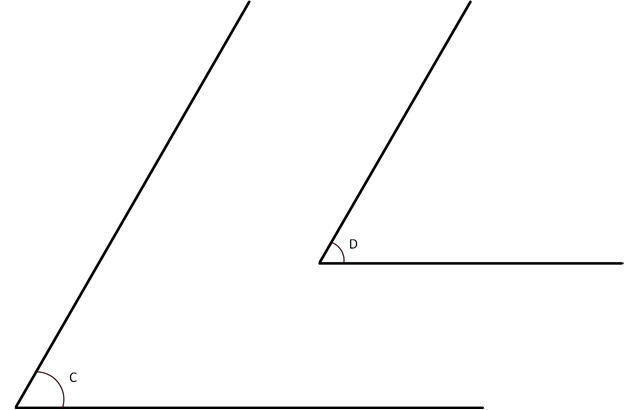Which angle is larger?  How do you know?

#### References

EngageNY Mathematics Grade 4 Mathematics > Module 4 > Topic B > Lesson 6Concept Development

Grade 4 Mathematics > Module 4 > Topic B > Lesson 6 of the New York State Common Core Mathematics Curriculum from EngageNY and Great Minds. © 2015 Great Minds. Licensed by EngageNY of the New York State Education Department under the CC BY-NC-SA 3.0 US license. Accessed Dec. 2, 2016, 5:15 p.m..

Modified by Fishtank Learning, Inc.

### Problem 3

What is the measure of the following angle?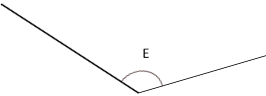## Problem Set & Homework

### Discussion of Problem Set

• Look at #1. How did you determine the angle measure without using a protractor? How can this skill help you when using an actual protractor to measure?
• In #2, which angle had the same measure as ${\angle G}$${\angle I}$?
• In #2, which angles had the same angle measure but different side length measures?
• For #3, discuss your experience of measuring with different protractors. Describe how the length of an arc on each protractor did or did not affect the measure of the given angle.
• Take a look at your 180° protractor. Find pairs of numbers that label the two scales, such as 150° and 30°. Name other pairs of numbers. What do you notice about the pairs of numbers?

?

First, estimate the measure of each angle below. Then, use a protractor to find the measure, in degrees, of each angle below.

a.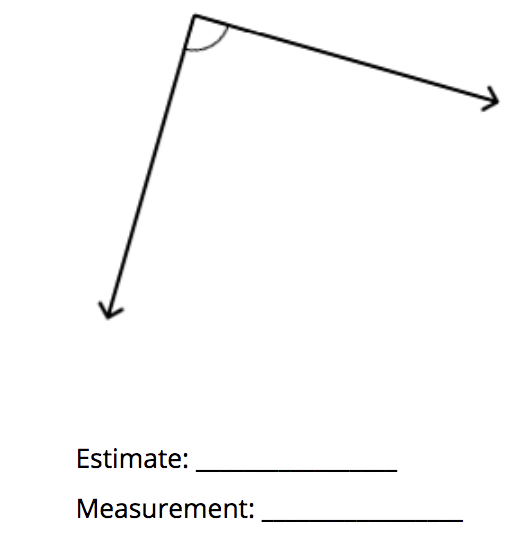b.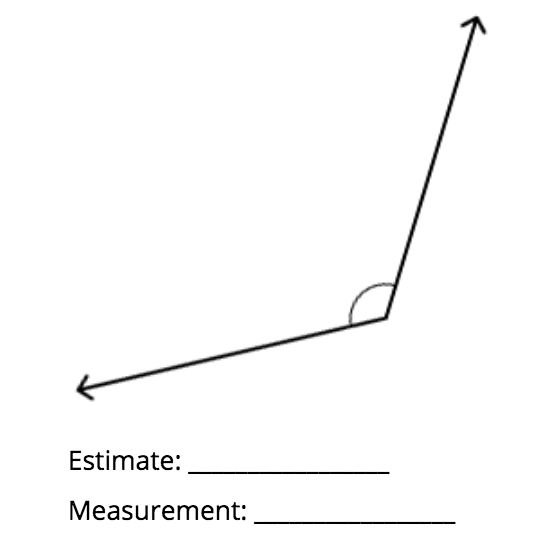c.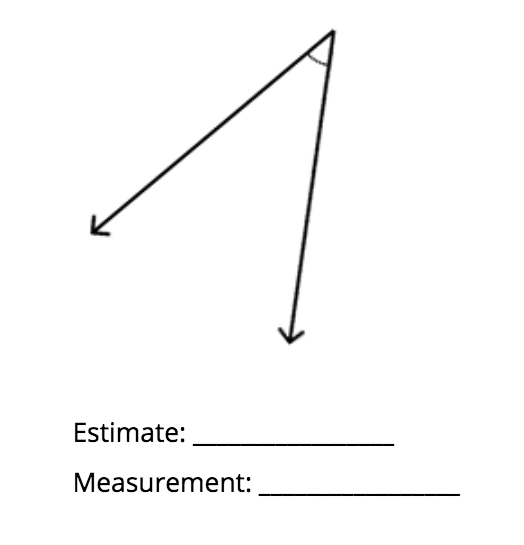d.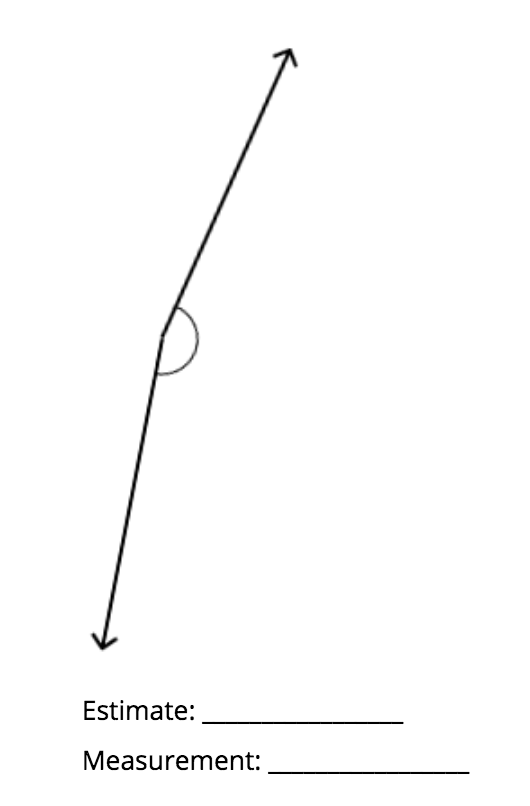#### Mastery Response